Skip to main content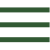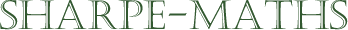Previous topic: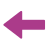Current topic:
Equivalent fractions & cancelling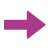Next topic:
Mixed numbers & top heavy fractions

### Equivalent fractions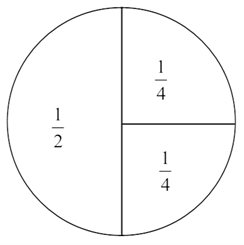Equivalent fractions are fractions which mean exactly the same thing but which are written in a completely different form.  In the diagram below you can see a circle split into halves and then into quarters.  We can see here that one half takes up exactly the same area as two quarters. This means that if I have one half of a pizza and my friend has two quarters of a pizza, we have exactly the same amount of pizza.

${1 \over 2} = {2 \times {1 \over 4}} = {2 \over 4}$

Now every fraction has an infinite number of equivalent fractions, so we will not want to list them all, as this will take quite a while, but it does mean that we can always choose a convenient equivalent fraction when we need one. More of this later.

Calculations with equivalent fraction are pretty straight forward, so long as you remember this simple rule:

If you multiply or divide the numerator by a number, you must do exactly the same to the denominator, and vice versa in order to keep the same value.